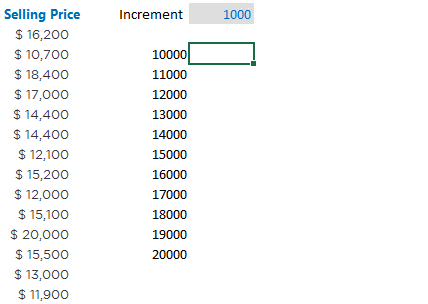The word ‘Class Interval‘ takes me back to the math class in school. Trust me, I had a tough time understanding Class Intervals and Frequency Distribution jargons. Several years later (now) when I finally believe I have understood its meaning and application, I want to keep it really simple for you and talk about 3 different ways to create it in Excel

All English and no Jargons.. promise!

###We have 100 transactions of products being sold. A typical question could be to create price bands to find how many transactions happened between each range. Something like this..Let me show 3 different ways to do this

### 1. Pivot Table Method1. Create a Pivot Table with the Data
2. Drop Product Sold in Values
3. Selling Price in Rows

Now let’s create price bands (class intervals)

We are going to use the grouping feature of Pivot Tables1. Right click on Price and Choose Group
2. Start at 10,000 and End at 20,000
3. The increment of 1,000

And we are done!

### 2. The Frequency Formula1. I am first selecting the range where the formula is supposed to be written. That is necessary because the formula is working on the entire range at once
2. The Frequency Formula has 2 parts
• Data Array – Where the actual data lies. In our case it will be the Selling Price
• Bins Array – Think of Bins as price range buckets. I’ll talk about this in detail in a moment
3. Since this is an array formula, use CTRL SHIFT ENTER to confirm the formula
4. Take a note that 100 records tally with the total

If you compare the results of the Frequency Formula with Pivot Table the results, they will vary a bit. For instance the values between 11k – 11,999 are not same as 12k range in frequency formulaThis is how the frequency formula reads the ranges and that is why the results vary a bit### 3. Countifs FormulaNote a few things

1. The result of the Countifs formula is the same as Frequency Formula
2. The criteria has been put in double quotes (” “) that is mandatory in the Countif/S function